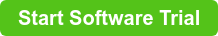v9.5 introduces decision tables, a powerful way of storing and using rules in workflows.  We gave an introduction to decision tables, but let's discuss more complex decision tables in conjunction with expressions.

Simple examples pass in input values and they are matched with the decision table inputs, but decision tables also support more complex expressions, such as passing an input value and using the value in expressions.   Below is a decision table that uses many input expressions to determine the risk value:As you can see, the input uses the input value to be evaluated as an expression to determine the output risk values.  This is only a simple example of using evaluated expressions in decision tables, but expressions can be complex as you want.

As we introduce this new decision table in v9.5, with our customer input, decision tables will evolve over time. With out of the box functionality in decision tables, much can be accomplished.  Decision tables are also fully accessible through the .Net api as well.